﻿ Recurrence Relations for Moments of k-th Upper Record Values from Flexible Weibull Distribution and a Characterization

### Recurrence Relations for Moments of k-th Upper Record Values from Flexible Weibull Distribution and ...

Mahmoud Ali Selim, Hamdy M. SalemOPEN ACCESSPEER-REVIEWED

## Recurrence Relations for Moments of k-th Upper Record Values from Flexible Weibull Distribution and a Characterization

Mahmoud Ali Selim1,, Hamdy M. Salem2

1Department of Statistics, Faculty of Commerce, Al-Azher University, Egypt & King Khalid University, Community College, Saudi Arabia

2Department of Statistics, Faculty of Commerce, Al-Azher University, Egypt & Qassim University, Community College in Buraidah, Saudi Arabia

### Abstract

In this paper, we establish some recurrence relations satisfied by single and product moments of k-th upper record values from the flexible Weibull distribution. We also give a characterization of flexible Weibull distribution by using the recurrence relations for single moments.

• Selim, Mahmoud Ali, and Hamdy M. Salem. "Recurrence Relations for Moments of k-th Upper Record Values from Flexible Weibull Distribution and a Characterization." American Journal of Applied Mathematics and Statistics 2.3 (2014): 168-171.
• Selim, M. A. , & Salem, H. M. (2014). Recurrence Relations for Moments of k-th Upper Record Values from Flexible Weibull Distribution and a Characterization. American Journal of Applied Mathematics and Statistics, 2(3), 168-171.
• Selim, Mahmoud Ali, and Hamdy M. Salem. "Recurrence Relations for Moments of k-th Upper Record Values from Flexible Weibull Distribution and a Characterization." American Journal of Applied Mathematics and Statistics 2, no. 3 (2014): 168-171.

 Import into BibTeX Import into EndNote Import into RefMan Import into RefWorks

### 1. Introduction

Chandler (1952) was the first to introduce the concept of record values and record statistics. Let {Xn, n≥1} be a sequence of independent and identically distributed random variables with a cumulative distribution function F(x) and probability density function f(x). An observation Xj will be called an upper record value if its value exceeds all previous observations. Thus, Xj is an upper record value if Xj> Xi for every i<j. For a fixed positive integer k, Dziubdziela and Kopocinski (1976) defined the sequence {Un(k), n≥1}of k-th upper record times for the sequence {Xn, n≥1}as follows: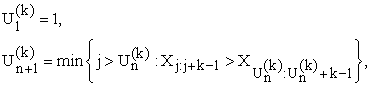Where Xj:m is the j-th order statistic of the sample X1, X2,…, Xm. Then the sequence {Yn(k), n≥1} where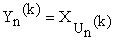is called a sequence of k-th upper record values of {Xn, n≥1}. Note that for k = 1, we get the usual upper record values as defined in Chandler (1952). For convenience, we also take Y0(k)=0 and Y1(k)=min(X1, X2,…, XN).

Recently, a new two-parameter ageing distribution called a flexible Weibull distribution was proposed by Bebbington et al. (2006) as a generalization of Weibull distribution. The probability density function (pdf) of the flexible Weibull distribution is given by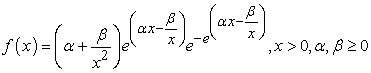(1.1)

and the corresponding survival function is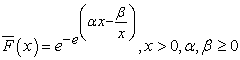(1.2)

where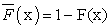. Note that for β = 0, this distribution reduces to type 1 extreme value distribution, see Johnson et al. (1995). Bebbington et al. (2006) shown that the flexible Weibull distribution is quite flexible, being able to model various ageing classes of lifetime distributions. So we can say that the flexible Weibull distribution is very important in several basic fields include engineering sciences, reliability, biological, demography and actuarial sciences. For more details on the statistical properties and estimation procedures of this distribution, one may refer to Bebbington et al. (2006), Habib et al. (2012) and Singh et al. (2013). Notation FWD(α,β) is used to denote the flexible Weibull distribution with two parameters α,β.

The moments of k-th record values have received considerable attention in the recent years. Many authors derived the recurrence relations for k-th record values for different distributions; See, Pawlas and Szynal (1999, 2000), Saran and Singh (2008), Bieniek and Szynal (2002, 2007, 2013), Nain (2010), Selim(2011), Kumar (2011, 2012), Kumar and Khan (2012) and Kumar and Kulshrestha (2013). The recurrence relations for moments of k-th record values from flexible Weibull distribution have not been considered in the earlier literature. In Section 2, some recurrence relations for the single and product moments of k-th upper record values from the flexible Weibull distribution are derived. In Section 3, a characterization of the flexible Weibull distribution is obtained by using a recurrence relation for single moments. Finally, conclusions are given in Section 4.

We shall denote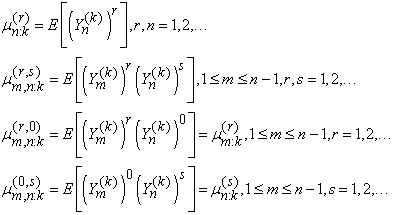### 2. Recurrence Relations for Single and Product Moments

The relation between pdf and survival function of FWD(α,β) in (1.1) and (1.2), respectively, can be written in the form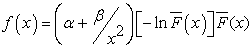(2.1)

This relation will be used in this paper to derive some recurrence relations for the single and product moments of k-th upper record values from FWD(α,β).

Let {Yn(k), n≥1}, wherebe a sequence of k-th upper record values arising from FWD(α,β). Then the pdf of Yn(k), n≥1 (see Dziubdziela and Kopocinski, (1976)) is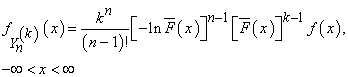(2.2)

and the joint pdf of Ym(k) and Yn(k), 1≤m<n, n≥2 is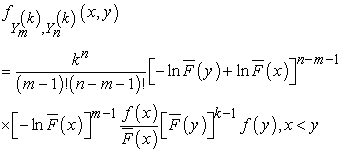(2.3)

Now, the recurrence relations for single and product moments of k-th upper record values from the flexible Weibull distribution can be introduced in the following theorems.

Theorem 1

For n≥1 and r=2,3,…,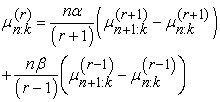(2.4)

and for n=1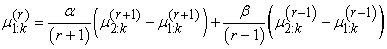(2.5)

For n≥2 and r=0,1,2,…,. Using the pdf of XU(n) given in (2.2) and the relation in (2.1), we have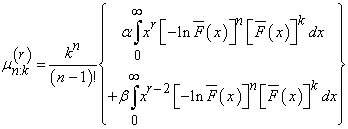(2.6)

Integrating by parts treating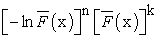for differentiation and the rest of the integrand for integration, we obtain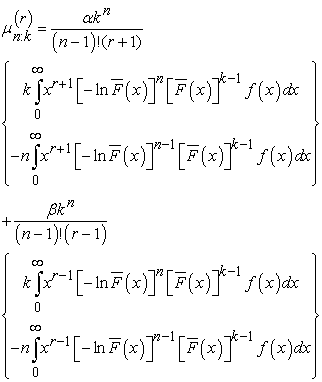(2.7)

Upon rewriting the above expression, we immediately obtain the relation (2.4).

Also, the relation (2.5) follows from (2.7) by setting n=1.

Remarks 1

(a) Putting k=1, in (2.4) and (2.5) we can deduce the recurrence relations for single moments of the usual upper record values from the flexible Weibull distribution.

(b) Putting β=0 in (2.4) and simplifying, we get the recurrence relation for single moments of k-th upper record values from type 1 extreme value distribution as follow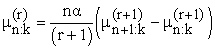(2.8)

Theorem 2

For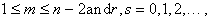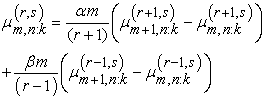(2.9)

and for m≥1 and r,s=0,1,2,…,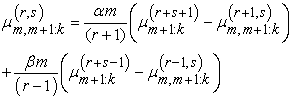(2.10)

Proof

For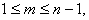and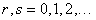using (2.3) and (2.1), we have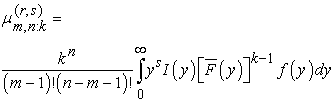(2.11)

where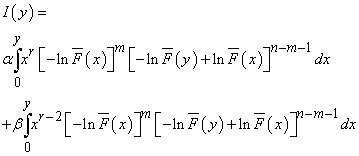Integrating I(y) by parts, treating xr for integration and the rest of the integrand for differentiation in the first term and in the second term treating xr-2 for integration and the rest of the integrand for differentiation, we obtain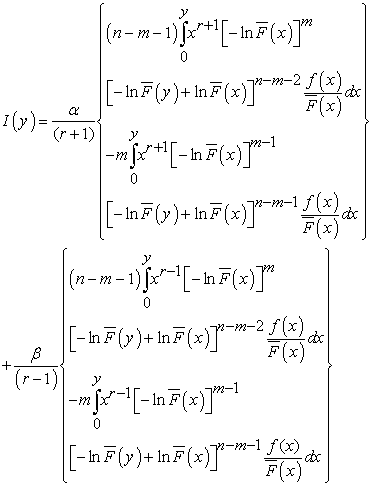Substituting the above expression in to (2.11) and simplifying, it leads to equation (2.9). And when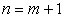, then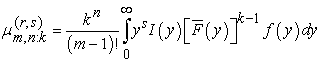(2.12)

Where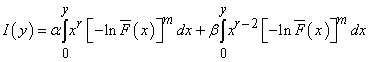Integrating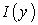by parts, treating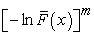for differentiation and the rest of the integrand for integration we get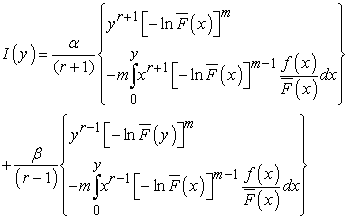Substituting the above expression in to (2.12) and simplifying, we obtain (2.10).

Remarks 2

(a) Putting k= 1, in (2.9) and (2.10) we deduce the recurrence relations for product moments of usual upper record values from the flexible Weibull distribution.

(b) Putting β = 0, in (2.9) and simplifying, we get the recurrence relation for product moments of k-th upper record values from type 1 extreme value distribution as follow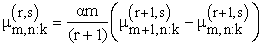(2.13)

### 3. A Characterization of the Flexible Weibull Distribution

In this section, we present a characterization for flexible Weibull distribution using the relation in (2.4) based on the following result of Lin(1986).

Proposition 1

Let n0 be any fixed non-negative integer and let a,b be real numbers such that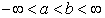. Let g(x)≥0 be an absolutely continuous function with g’(x)≠0 almost everywhere on (a,b). Then the sequence of functions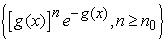is complete in L(a,b)if and only if g(x) is strictly monotone on (a,b).

Theorem 3

For a fixed positive integer k. A necessary and sufficient condition for a random variable X to be distributed with probability density function of the flexible Weibull distribution given by (1.1) is that(3.1)

Proof

The necessary part follows immediately from (2.4), on the other hand if the recurrence relation in (3.1) is satisfied, then on rearranging the terms in (3.1) and using (2.2), we have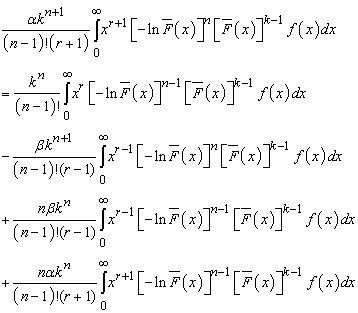(3.2)

Integrating the last two integrals on the right hand side of (3.2) by parts, we get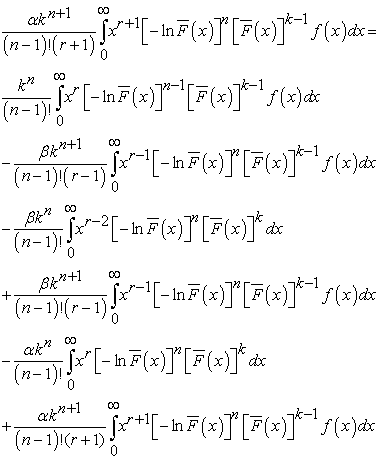(3.3)

Upon simplification the above expression, we obtain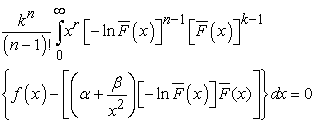(3.4)

Using the proposed 1, we conclude that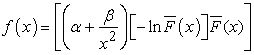This proves that f(x) is probability density function of the flexible Weibull distribution.

### 4. Conclusions Remarks

(1). In this paper, some recurrence relations for single and product moments of k-th upper record values from flexible Weibull distribution have been derived.

(2). The recurrence relation for single moments of k-th upper record values has been utilized to obtain a characterization of flexible Weibull distribution.

(3). The recurrence relations for moments of k-records are important because they can be helpful in reducing the amount of direct calculations needed to calculate the moments. And they can be used in a simple recursive manner to express the unknown higher order moments in terms of lower order moments thus making the evaluation of higher moments easy. Also they can be used to characterize the distributions.

### References

  Bebbington, M., Lai, C.D. and Zitikis, R. (2006). A flexible Weibull extension, Reliability Engineering and System Safety, 92, 719-726.In article CrossRef  Bieniek, M., and Szynal, D. (2002). Recurrence relations for distribution functions and moments of k-th Record values, Journal of Mathematical Sciences, 111, 3511-3519.In article CrossRef  Bieniek, M., and Szynal, D. (2007). On k-th record times, record values and their moments, Journal of Statistical Planning and Inference, 137, 12-22.In article CrossRef  Bieniek, M., and Szynal, D. (2013). On moments of k-th record values from the linear exponential distribution. Journal of mathematical sciences, 191 (4), 526-537.‏In article CrossRef  Chandler, K.N. (1952). The distribution and frequency of record values, Journal of Royal Statistical Society, B, 14, 220-228.In article  Dziubdziela, W., and Kopocinski, B. (1976). Limiting properties of the k-th record value, Zastosowania Mathematyki, 15, 187-190.In article  Habib, M.E., Shams, T.M., Selim, M.A. and Hussen, A.A. (2012). Maximum likelihood estimation of the flexible Weibull distribution parameters based on type I censored data. Academy of Business Journal, Al-Azhar University, 9, 1, pp 50-70.In article  Johnson, N. L., Kotz, S., and Balakrishan, N. (1995). Continuous Univariate Distributions (2nd ed., Vol. 2). New York: Wiley.In article  Kumar, D. (2011). Recurrence relations for moments of k-th lower record values from exponentiated log-logistic distribution and a characterization, International Journal of Mathematical Archive, 2, 6, 813-319.In article  Kumar, D. (2012): Recurrence relations for marginal and joint moment generating functions of generalized logistic distribution based on lower k record values and its characterization. ProbStat Forum, 5, 47-53.In article  Kumar, D. and Khan, M.I. (2012): Recurrence relations for moments of k th record values from generalized beta distribution and a characterization. Selçuk Journal of Applied Mathematics. 13, 75-82.In article  Kumar, D., and Kulshrestha, A. (2013). Expectation Identities of Upper Record Values from Generalized Pareto Distribution and a Characterization. Journal of Statistics Applications & Probability, 2 (2), 115-121.‏In article CrossRef  Lin, G.D. (1986). On a moment problem, Tohoku Math. J., 38, 595-598.In article CrossRef  Nain, K. (2010). Recurrence relations for single and product moments of k th record values from generalized Weibull distribution and a characterization. International Mathematical Forum, 5, 33, 1645-1652.‏In article  Pawlas, P., and Szynal, D. (1999). Recurrence relations for single and product moment of k-th record values from Pareto, generalized Pareto and Burr distributions. Communication in Statistics-Theory and Methods, 28, 7, 1699-1709.In article CrossRef  Pawlas, P., and Szynal, D. (2000). Recurrence relations for single and product moment of k-th record values from Weibull distribution and a characterization, Journal of Applied Statistical Science, 10, 17- 25.In article  Saran, J., and Singh, S.K. (2008). Recurrence relations for single and product moments of k-th record values from linear exponential distribution and a characterization. Asian J. Math. Stat, 1, 159-164.‏In article CrossRef  Selim, M.A. (2011). Recurrence relations for single and product moments of k-th record values from modified Weibull distribution and a characterization. Academy of Business Journal, AL-Azhar University, 8, 1, pp 75-87.In article  Singh, S.K., Singh, U., and Sharma, V.K. (2013). Bayesian estimation and prediction for flexible Weibull model under type-II censoring scheme. Journal of Probability and Statistics, 2013.‏In article